# Sparse Matrix using Linked List in C

## Sparse Matrix using Linked List in C Language:

In this article, I am going to discuss Sparse Matrix using Linked List in C Language with Examples. Please read our previous article, where we discussed How to Perform Insertion in a Doubly Linked List using C Language with Examples.

##### Sparse Matrix using Linked List in C Language:

Now, we will discuss the representation of a sparse matrix, and also, we will see how to display a sparse matrix from that representation. We have already studied this topic in arrays. Now we are learning it in the linked list. We have an example of a sparse matrix of size 5×6 i.e. 5 rows and 6 columns.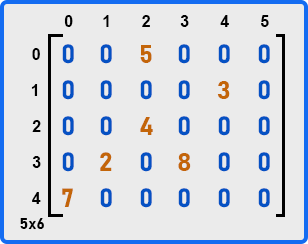Here a lot of elements are zeroes and some are non-zeroes elements i.e. 5, 3, 4, 2, 8, and 7. How to represent these elements?

##### Representation of Sparse Matrix:

To represent a sparse matrix, we want to avoid storing zeroes. We want to store only non-zero elements. For example, if we take 5, this is a non-zero element. So, for representing it, we should know the non-zero element and also the position of that element in the matrix. For that, we should know the row and column numbers for non-zero elements. 5 is present at (0, 2) location. Similarly following are the locations of non-zero elements.

5 – (0, 2)
3 – (1, 4)
4 – (2, 2)
2 – (3, 1)
8 – (3, 3)
7 – (4, 0)

So, we have to represent this data in such that we should be able to regenerate the same sparse matrix. So let us see how we can represent this one by just taking non-zero elements.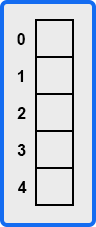For representation, we have taken an array. This array will represent rows. As our matrix has 5 rows so we have taken an array of size 5. The indices of this array represent the row number of the matrix. Now for representing non-zero elements, as the array represents the row number so we just need to know the column number and the element itself. Let us say the element is 5 and the column number is 2. So, this we will represent as a node.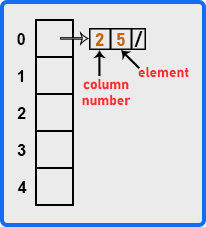Here 0th row has a node that contains column number (2), non-zero element (5), and a pointer to the next node (here that is null ‘/’). Now let us add all the non-zero elements according to their row number in the above array.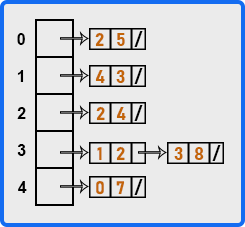The above diagram represents all the non-zero elements of the given matrix. Let us say it is array A. So we can say that A is an array of linked lists. Now, how we can form this structure? This structure is having a collection of linked lists and a linked list is a collection of nodes, so each node contains a column number and a non-zero element. So let us define the node structure,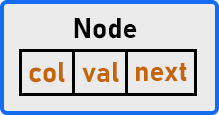The node structure contains 3 things that are column number, a non-zero element, and a pointer to the next node (if any is otherwise null). We can define the structure of this node in C language as,

struct Node{
int col;
int val;
struct Node *next;
}

Now how to create the structure for storing only non-zero elements? For creating a structure, we need an array of the number of rows size. If the matrix is of order m x n, we will create an array of size m.

##### Display of Sparse Matrix:

Let us write the pseudo-code for displaying a sparse matrix.

```for(i = 0; i < m; i++){
p = A[i];
for(j = 0; j < n; j++){
if(j == p->col){
printf("%d", p->val);
p = p->next;
}
else{
printf("0");
}
}
}
```
##### Example to understand Sparse Matrix using C language:
```#include <stdio.h>
#include <stdlib.h>

struct Node {
int column;
int element;
struct Node *next;
};

void CreateSparseMatrix(struct Node **X){
int row, col, ele;
struct Node *p;
scanf("%d %d %d", &row, &col, &ele);
if(X[row] == NULL){
X[row] = (struct Node *) malloc(sizeof(struct Node));
p = X[row];
p->column = col;
p->element = ele;
p->next = NULL;
}
else{
p = X[row];
while(p->next != NULL){
p=p->next;
}
struct Node *temp = (struct Node *) malloc(sizeof(struct Node));
temp->column = col;
temp->element = ele;
temp->next = NULL;
p->next = temp;
}
}

void DisplaySparseMatrix(struct Node **X, int m, int n){
for(int i = 0; i < m; i++){
struct Node *p = X[i];
for(int j = 0; j < n; j++){
if(p == NULL){
printf("0 ");
}
else if(j == p->column){
printf("%d ", p->element);
if(p->next != NULL)
p = p->next;
}
else{
printf("0 ");
}
}
printf("\n");
}
}

int main(){
int m, n, x;
printf("Enter matrix dimensions:");
scanf("%d %d", &m, &n);
printf("Enter total number of non-zero elements: \n");
scanf("%d", &x);

struct Node** A = (struct Node **) malloc(sizeof(struct Node) * m);

printf("Enter all non-zero Elements:\n");
for(int i = 1; i <= x; i++){
printf("Enter row number, column number and non-zero element:\n");
CreateSparseMatrix(A);
}

printf("\nSparse Matrix: \n");
DisplaySparseMatrix(A, m, n);
}
```
###### Output: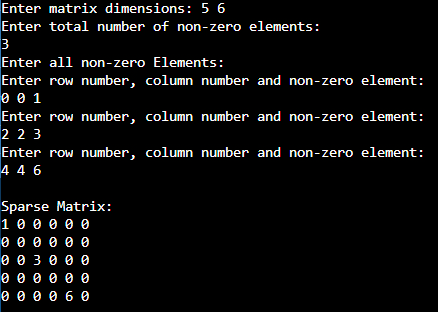In the next article, I am going to discuss Polynomial Representation using Linked List in C Language. Here, in this article, I try to explain Sparse Matrix using Linked List in C Language with Examples. I hope you enjoy this Sparse Matrix using Linked List in the C Language article